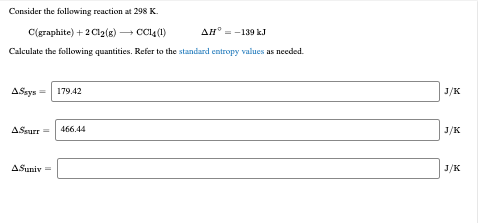Question

# Data: (1) As(s) + 3/2Cl2(g) -> AsCl3(g)   ΔH = -261.5 kJ (2) As(s) + 3/2Cl2(g) ->...

Data:

(1) As(s) + 3/2Cl2(g) -> AsCl3(g)   ΔH = -261.5 kJ

(2) As(s) + 3/2Cl2(g) -> AsCl3(l)   ΔH = -305.1 kJ

On the basis of the above data, which of the following statements is false?

1. ΔH for the reaction AsCl3(l) -> AsCl3(g) is 43.6 kJ

2. Reaction (2) is the formation reaction for AsCl3(g)

3. The energy content in AsCl3(l) is lower than AsCl3(g)

4. Reaction (1) is exothermic

5. The reverse of reaction (2) is endothermic

(2) is false statement.

It is the formation reaction for AsCl3(l).

So it is false

#### Earn Coins

Coins can be redeemed for fabulous gifts.

Similar Homework Help Questions
• ### Consider the following reaction at 298 K. C(graphite)+2Cl2(g)⟶CCl4(l)ΔH∘=−139 kJC(graphite)+2Cl2(g)⟶CCl4(l)ΔH∘=−139 kJ Calculate the following quantities. Refer to...Consider the following reaction at 298 K. C(graphite)+2Cl2(g)⟶CCl4(l)ΔH∘=−139 kJC(graphite)+2Cl2(g)⟶CCl4(l)ΔH∘=−139 kJ Calculate the following quantities. Refer to the standard entropy values as needed. Consider the following reaction at 298 K. C(graphite) + 2Cl2(g) C014 (1) AH = -139 kJ Calculate the following quantities. Refer to the standard entropy values as needed. A\$sys = 179.42 ASsurr = 466.44 A.Sunix =

• ### Hess's Law Given the following data: P(s) + 3/2H2(g) → PH3(g) ΔH°= +5.0 kJ PH3(g) +...

Hess's Law Given the following data: P(s) + 3/2H2(g) → PH3(g) ΔH°= +5.0 kJ PH3(g) + 2O2(g) → H3PO4(l) ΔH°= -1272.0 kJ 2P(s) + 5/2O2(g) → P2O5(s) ΔH°= -1492.0 kJ H2(g) + 1/2O2(g) → H2O(l) ΔH°= -286.0 kJ calculate ΔH° for the reaction: H3PO4(l) → 1/2P2O5(s) + 3/2H2O(l) ΔH°=?? also please explain how you found the answer

• ### Consider the reaction: H2(g) + (1/2)O2(g) -------> H2O(l) ΔH° = -286 kJ Which of the following...

Consider the reaction: H2(g) + (1/2)O2(g) -------> H2O(l) ΔH° = -286 kJ Which of the following is true? (Select all that apply) the reaction is endothermic heat is given off by the surroundings the reaction is exothermic heat is absorbed by the system the enthalpy of the products is less than the that of the reactants

• ### The heat of formation(ΔHfO) for AsCl3(l) is -305.0 kJ/mol, and ΔHO = -548.8 kJ for the...

The heat of formation(ΔHfO) for AsCl3(l) is -305.0 kJ/mol, and ΔHO = -548.8 kJ for the following reaction: 2 As (s) + 3 Cl2 (l) --> 2 AsCl3 (l) Find the heat of vaporization for Cl2. (Vaporization is a phase change from liquid to gas, so the heat of vaporization would be ΔHO for the process Cl2(l) --> Cl2(g).)

• ### Given the following data: C(s) + O2(g) → CO2(g) ΔH = -393.5 kJ CS2(l) + 3O2(g) → CO2(g) + 2SO2(g) ΔH = -1076.5 kJ C(s) +...

Given the following data: C(s) + O2(g) → CO2(g) ΔH = -393.5 kJ CS2(l) + 3O2(g) → CO2(g) + 2SO2(g) ΔH = -1076.5 kJ C(s) + 2S(s) → CS2(l) ΔH = +89.4 kJ Find the ΔH of the following reaction: SO2(g) → S(s) + O2(g)

• ### Consider the following data. 2 H2(g) + O2(g) 2 H2O(l) ΔH = -571.7 kJ N2O5(g) +...

Consider the following data. 2 H2(g) + O2(g) 2 H2O(l) ΔH = -571.7 kJ N2O5(g) + H2O(l) 2 HNO3(l) ΔH = -92.0 kJ N2(g) + 3 O2(g) + H2(g) 2 HNO3O(l) ΔH = -348.2 kJ Use Hess's law to calculate ΔH for the reaction below. 2 N2O5(g) 2 N2(g) + 5 O2(g) ΔH = _____kJ

• ### 1. The dimensions of the SI unit of energy, the Joule (J), are A. K B....

1. The dimensions of the SI unit of energy, the Joule (J), are A. K B. kg m s C. kg m2 s-2 D. kg m-1 s-2 E. kg m2 s-1 2. Natural gas, or methane, is an important fuel. Combustion of one mole of methane releases 802.3 kilojoules of energy. How much energy does that represent in kilocalories? A. 3.36×103 kcal B. 3.357 kcal C. 191.8 kcal D. 0.192 kcal E. 1.918×105 kcal 3. What is the change in...

• ### a) Use Hess's law to calculate the enthalpy change for the reaction: 3C(s) + 4H2(g) +...

a) Use Hess's law to calculate the enthalpy change for the reaction: 3C(s) + 4H2(g) + ½O2(g) → C3H8O(l) Given the following thermochemical equations: 2C3H8O(l) + 9O2(g) → 6CO2(g) + 8H2O(l)    ΔH = -4042.6 kJ/mol C(s) + O2(g) → CO2(g)    ΔH = -393.51 kJ/mol H2(g) + ½O2(g) → H2O(l)    ΔH = -285.83 kJ/mol (in kJ/mol) A: -267.7 B: -302.6 C: -341.9 D: -386.3 E: -436.5 F: -493.3 G: -557.4 H: -629.9 b) Define if the following statement is an endothermic process or exothermic...

• ### You are given the following thermodynamic data. 2 Fe(s) + 3/2 O2(g) → Fe2O3(s) ΔH° =...

You are given the following thermodynamic data. 2 Fe(s) + 3/2 O2(g) → Fe2O3(s) ΔH° = -823 kJ 3 Fe(s) + 2 O2(g) → Fe3O4(s) ΔH° = -1120. kJ Calculate the ΔH° for the following reaction. 3 Fe2O3(s) → 2 Fe3O4(s) + ½ O2(g)

• ### ΔH for the reaction IF_{5}5​(g) → IF_{3}3​(g) + F_{2}2​ is kJ, give the data below. IF(g)...

ΔH for the reaction IF_{5}5​(g) → IF_{3}3​(g) + F_{2}2​ is kJ, give the data below. IF(g) + F_{2}2​(g) → IF_{3}3​(g) ΔH = -390 kJ IF(g) + 2F_{2}2​(g) → IF_{5}5​(g) ΔH = -745 kJ